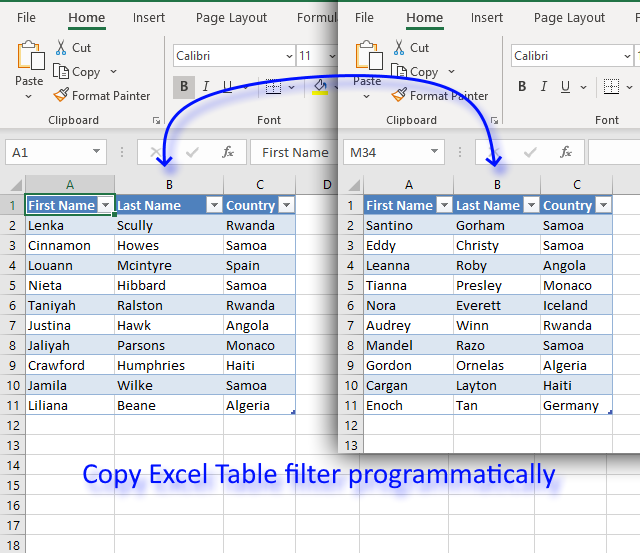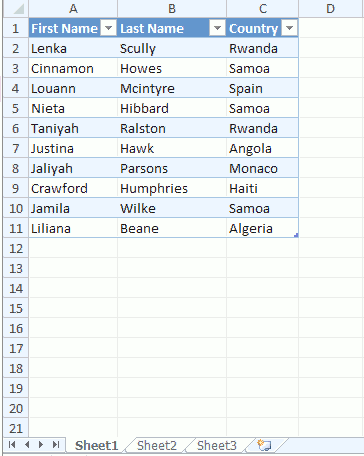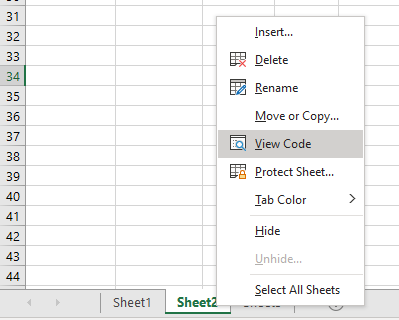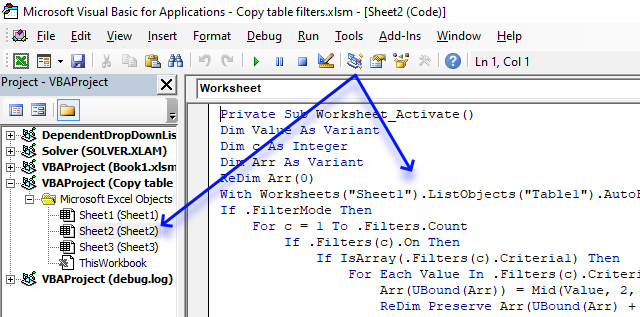Author: Oscar Cronquist Article last updated on October 08, 2019I will in this article demonstrate a macro that copies criteria from one Excel Table and applies them to another Excel Table.

### How it worksThe animated image above shows selecting criteria manually in one Excel Table. An event macro then automatically copies the criteria to another table located on a different worksheet when that worksheet is activated.

It is required that both tables have the same column header names and the columns are arranged in the same order.

### VBA code

```'Event code located at a worksheet module
Private Sub Worksheet_Activate()

'Dimension variable and declare data types
Dim Value As Variant
Dim c As Integer
Dim Arr As Variant

'Redimension array variable Arr in order to programmatically increase array size
ReDim Arr(0)

'The With ... End With statement allows you to write shorter code by referring to an object only once instead of using it with each property.
With Worksheets("Sheet1").ListObjects("Table1").AutoFilter

'Check if FilterMode is True
If .FilterMode Then

'Iterate through filters
For c = 1 To .Filters.Count

'Check if filter is on
If .Filters(c).On Then

'Check if filter contains multiple conditions
If IsArray(.Filters(c).Criteria1) Then

'Iterate through each criteria value
For Each Value In .Filters(c).Criteria1

'Save criteria value to array variable Arr
Arr(UBound(Arr)) = Mid(Value, 2, Len(Value))

'Increase array size with 1
ReDim Preserve Arr(UBound(Arr) + 1)

'Continue with next criteria value
Next

'Decrease array size with 1
ReDim Preserve Arr(UBound(Arr) - 1)

'Excel continues here if filter contains a single condition
Else

'Save criteria value to array variable Arr
Arr(UBound(Arr)) = .Filters(c).Criteria1

'Increase size of array variable Arr
ReDim Preserve Arr(UBound(Arr) + 1)

'Enable error handling
On Error Resume Next

'Save criteria value to array variable Arr
Arr(UBound(Arr)) = .Filters(c).Criteria2

'Check if an error has occured then decrease array size with 1
If Err <> 0 Then ReDim Preserve Arr(UBound(Arr) - 1)

'Disable error handling
On Error GoTo 0

'Exit If statement
End If

'Enable Excel Table filter for Table2
Worksheets("Sheet2").ListObjects("Table2").Range.AutoFilter Field:=c

'Apply Excel Table filter criteria for Table2 based on variable c
Worksheets("Sheet2").ListObjects("Table2").Range.AutoFilter Field:=c, Criteria1:=Arr, Operator:=xlFilterValues
End If
Next
Else

'Iterate
For c = 1 To ActiveSheet.ListObjects("Table2").Range.Columns.Count
ActiveSheet.ListObjects("Table2").Range.AutoFilter Field:=c
Next
End If
End With
End Sub
```

### Where to put the code?

1. Copy above VBA code.
2. Press with right mouse button on on tab sheet2, you will find it at the very bottom of the Excel screen.3. Press with left mouse button on "View Code".
4. Paste VBA code to sheet module.